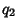## q-Analog

A-analog, also called a q-Extension or q-Generalization, is a mathematical expression parameterized by a quantitywhich generalizes a known expression and reduces to the known expression in the limit. There are-analogs of the Factorial, Binomial Coefficient, Derivative, Integral, Fibonacci Numbers, and so on. Koornwinder, Suslov, and Bustoz, have even managed some kind of-Fourier analysis.

The-analog of a mathematical object is generally called the-object'', hence q-Binomial Coefficient, q-Factorial, etc. There are generally several-analogs if there is one, and there is sometimes even a multibasic analog with independent,, ....

See also d-Analog, q-Beta Function, q-Binomial Coefficient, q-Binomial Theorem, q-Cosine, q-Derivative, q-Factorial, q-Gamma Function, q-Series, q-Sine, q-Vandermonde Sum

References

Exton, H.-Hypergeometric Functions and Applications. New York: Halstead Press, 1983.